Help For: Functions for counting representations of quivers

Jiuzhao Hua

Function: numberofreps1,numberofreps2,numberofreps3 - compute numbers of representations of quivers over a finite field

Calling Sequence:

numberofreps1(a11,N)

numberofreps2(a11,a22,a12,N)

numberofreps3(a11,a22,a33,a12,a23,a31,N)

Parameters:

aij - number of edges between vertices i and j in a graph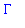N - upper bound for the entries of the dimension vector

Description:

This program computes the number of isomorphism classes of representations, indecomposable representations and absolutely indecomposable representations of a quiverwith up to three vertices over a finite field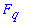.is a graph with up to three vertices.

These functions compute polynomials,andwithless than or equal to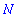simultaneously where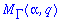is the number of isoclasses of representations of(with an arbitrary orientation) over\$ with dimension vector,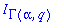is the number of isoclasses of indecomposable representations of(with an arbitrary orientation) overwith dimension vector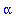,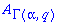is the number of isoclasses of absolutely indecomposable representations of(with an arbitrary orientation) overwith dimension vector.

For the function numberofreps1 to work properly, the integer N is less than or equal 16, for numberofreps2 N is less than or equal 6 and for numberofreps3 N is less than or equal 4.

Examples:

> numberofreps1(2,3);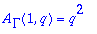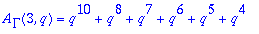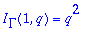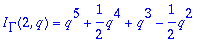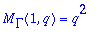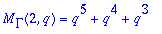Back to:
start page of the crep online manual